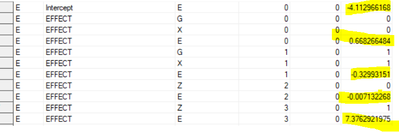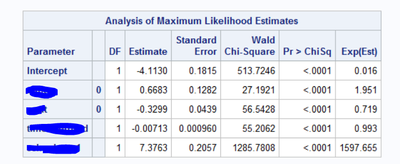## proc logistic inmodel

I use the following code to get regression coefficient

step 1

``````proc logistic data=d1 outmodel=outp_d1;
where   first=1;
class  examlevel sex/param=ref;
model pass (event="1")=capte sex time passrate/expb;
run;``````

Then I applied the regression coefficients got from step 1 to the equation and calculate probability for each examinee, as code below

step 2

``````data pre12;
set pre11;
if first=1 then logit=-3.9438+0.3341*examlevel-0.1650*sex-0.00713*time+7.3763*passrate;
run;

data pre12;
set pre12;
odds=exp(logit);
run;

data pre12;
set pre12;
prob_pred=odds/(1+odds);
run;``````

The other way to get probability is by using the results from step 1 as code below

``````proc logistic inmodel=outp_d1;
score clm data=pre11 out=prob_first;
where    first=1 ;
run;``````

On examinee level, I got (a little bit) higher probability by using plug in coefficients in the equation than using proc logistic inmodel statement. When I aggregate the probability on group level over thousands of examinees, the difference between these two method of calculate probability are close to 5%, which is to big to be ignored.  Any help would be much appreciated. Thanks!

13 REPLIES 13Ksharp
Super User

## Re: proc logistic inmodel

I think your code should like :

data pre12;
set pre12;
prob_pred=logistic(logit);
run;

And where is PRE11 from . You should include design matrix in it before getting/score predict probability .

## Re: proc logistic inmodel

Thank you very much for the help.

I tried prob_pred=logistic(logit) as you suggested to calculated the probability, I got the same probability as the calculation I used (step 2 in my original post) for each examinee.

My step 1 is for purpose of getting regression coefficients. Then I want to apply those coefficient to a dataset "pre11".  I manually plugged those coefficients and generated dataset "pre12" (as in step 2). Another method of applying those coefficients is using "proc logistic inmodel=" procedure (the bottom part of my original post). For each examinee, I got higher probability by manually plugging in coefficients than by using the proc logist inmodel procedure. The probability difference on group level is close to 5%. I have to find out where did the difference of probability come from between those two methods. I am wondering do you have any other suggestions for me to try? Thanks again!

## Re: proc logistic inmodel

The values you see in SAS tables are rounded values. So in Step 1, the value you typed in as -0.1650 might actually be -0.16495 or -0.165049. That is the most likely cause of your 5% error.

You can use the statement
ODS OUTPUT ParameterEstimates=OutP;

to write the parameter estimates to a SAS data set. You can then use

proc print data=outp;
format Estimate bestd16.;
run;

to see more precision for the estimates.

## Re: proc logistic inmodel

I tried the statements you suggested, but I can't see more decimal places in the output file after running proc print. My "outp" file looks like below, so I plugged the highlighted numbers in the equation. I randomly selected a few examinees, I noticed the probability for each examinee calculated by plugging in the coefficients is higher than using the "proc logistic inmodel=".

Any other suggestions for me to try?## Re: proc logistic inmodel

The picture you post is not the contents of the OUTP data set. It looks like the outp_d1 data set.

Copy/Paste the values of the ParameterEstimate table as text (not as an image). It will look something like this:

Obs Variable ClassVal0 DF Estimate StdErr WaldChiSq ProbChiSq ExpEst _ESTTYPE_
1 Intercept   1 0.847258 0.6901 1.5075 0.2195 2.333 MLE
2 Sex F 1 -0.624121 0.9624 0.4206 0.5167 0.536 MLE

## Re: proc logistic inmodel

Thank you for replying my question!

In my original post, as in the picture below (sorry for having to block the name of variables due to confidentiality), I copied and pasted the "Estimates" into the equation to calculate probability for each examinee, but got higher probability for each examinee than using "proc logist inmodel=" procedure to calculate probability. I hope I made my question clearer.## Re: proc logistic inmodel

I'll try one more time. Please do the following:

1. Run the following code:

``````proc logistic data=d1 outmodel=outp_d1;
where   first=1;
class  examlevel sex/param=ref;
model pass (event="1")=capte sex time passrate/expb;
ods output ParameterEstimates=OutP;
run;

proc print data=outp;
format Estimate bestd16.;
run;``````

2. Select the results of PROC PRINT. Copy them into the buffer. Paste them into your response. We are not interested in a screenshot image.

## Re: proc logistic inmodel

I tried as you suggested. The probabilities are still a little higher by coping and pasting the coefficients than using the proc logistic inmodel procedure. Is there anything we can do about proc logistic inmodel procedure? Or is there anything wrong of my proc logistic inmodel as below? Thank you!!

``````proc logistic inmodel=outp_d1;
score clm data=pre11 out=prob_first;
where    first=1 ;
run``````

## Re: proc logistic inmodel

Here's a way to show that the estimates are the same, any differences are typically from numerical precision issues.

https://communities.sas.com/t5/Statistical-Procedures/How-to-determine-logistic-regression-formula-f...

## Re: proc logistic inmodel

Thanks much for providing the information!

My sas code is as below

``````proc logistic data=d1 outmodel=outp_d1;
where   first=1;
class   sex (ref='0')/param=ref;
model pass (event="1")= sex time passrate/expb;
run;``````

in my code above, male is coded as 0. By using the code above, I got the following output, what does 1 (in bold) following sex mean? Does it mean 0.3299 is the parameter estimate for female? My results is a little bit counter intuitive. I'd like to check with you expert. Thank you for taking time to answer my question.

 Analysis of Maximum Likelihood Estimates Parameter DF Estimate StandardError WaldChi-Square Pr > ChiSq Exp(Est) Intercept 1 -3.7746 0.1433 693.8620 <.0001 0.023 sex 1 1 0.3299 0.0439 56.5428 <.0001 1.391 time 1 -0.00713 0.000960 55.2062 <.0001 0.993 pass 1 7.3763 0.2057 1285.7808 <.0001 1597.655

## Re: proc logistic inmodel

Yes, that is the estimate for when Female = 1
The estimate for Male ends up incorporated into the Intercept essentially, one of the effects of dummy coding.

If you're unsure of the interpretation use an explicit ODDSRATIO statement that lets you specify exactly what you want and see if the output matches what you expect.

## Re: proc logistic inmodel

Thank you all so much for your expertise!

I very much appreciate your time and help!

Discussion stats
• 13 replies
• 831 views
• 6 likes
• 4 in conversation